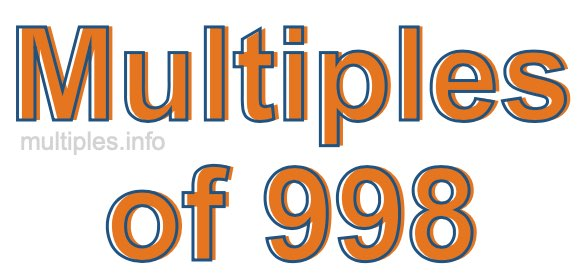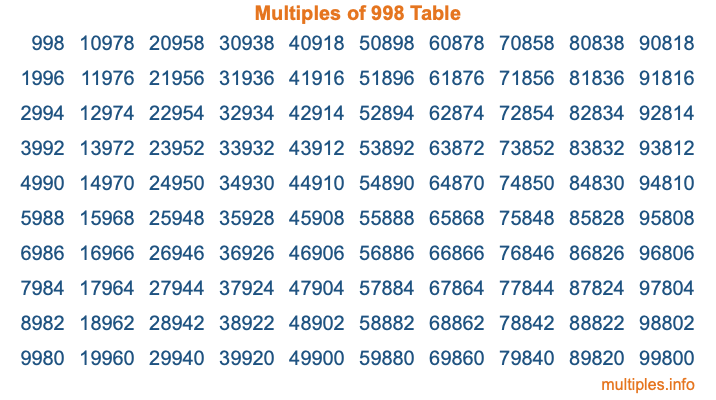Multiples of 998Welcome to the Multiples of 998 page. Here we will first teach you everything you will ever need to know about the multiples of 998, and then give you a study guide summary of everything we taught you to make sure you remember it all. Use this page to look up facts and learn information about the multiples of 998. This page will make you a multiples of nine hundred ninety-eight expert!

Definition of Multiples of 998
Multiples of 998 are all the numbers that when divided by 998 equal an integer. Each of the multiples of 998 are called a multiple. A multiple of 998 is created by multiplying 998 by an integer.

Therefore, to create a list of multiples of 998, you start with 1 multiplied by 998, then 2 multiplied by 998, then 3 multiplied by 998, and so on for as long as you want. Thus, the list of the first five multiples of 998 is 998, 1996, 2994, 3992, and 4990. To see a larger list of multiples of 998, see the printable image of Multiples of 998 further down on this page. We also have a category where you can choose any nth multiple of 998.

Multiples of 998 Checker
The Multiples of 998 Checker below checks to see if any number of your choice is a multiple of 998. In other words, it checks to see if there is any number (integer) that when multiplied by 998 will equal your number. To do that, we divide your number by 998. If the the quotient is an integer, then your number is a multiple of 998.

Is  a multiple of 998?

Least Common Multiple of 998 and ...
A Least Common Multiple (LCM) is the lowest multiple that two or more numbers have in common. This is also called the smallest common multiple or lowest common multiple and is useful to know when you are adding our subtracting fractions. Enter one or more numbers below (998 is already entered) to find the LCM.

Check out our LCM Calculator if you need more details about the Least Common Multiple or if you need the LCM for different numbers for adding and subtraction fractions.

nth Multiple of 998
As we stated above, 998 is the first multiple of 998, 1996 is the second multiple of 998, 2994 is the third multiple of 998, and so on. Enter a number below to find the nth multiple of 998.

th multiple of 998

Multiples of 998 vs Factors of 998
998 is a multiple of 998 and a factor of 998, but that is where the similarities end. All postive multiples of 998 are 998 or greater than 998. All positive factors of 998 are 998 or less than 998.

Below is the beginning list of multiples of 998 and the factors of 998 so you can compare:

Multiples of 998: 998, 1996, 2994, 3992, 4990, etc.

Factors of 998: 1, 2, 499, 998

As you can see, the multiples of 998 are all the numbers that you can divide by 998 to get a whole number. The factors of 998, on the other hand, are all the whole numbers that you can multiply by another whole number to get 998.

It's also interesting to note that if a number (x) is a factor of 998, then 998 will also be a multiple of that number (x).

Multiples of 998 vs Divisors of 998
The divisors of 998 are all the integers that 998 can be divided by evenly. Below is a list of the divisors of 998.

Divisors of 998: 1, 2, 499, 998

The interesting thing to note here is that if you take any multiple of 998 and divide it by a divisor of 998, you will see that the quotient is an integer.

Multiples of 998 Table
Below is an image of the first 100 multiples of 998 in a table. The table is in chronological order, column by column. The first column has the first ten multiples of 998, the second column has the next ten multiples of 998, and so on.The Multiples of 998 Table is also referred to as the 998 Times Table or Times Table of 998. You are welcome to print out our table for your studies.

Negative Multiples of 998
Although not often discussed or needed in math, it is worth mentioning that you can make a list of negative multiples of 998 by multiplying 998 by -1, then by -2, then by -3, and so on, to get the following list of negative multiples of 998:

-998, -1996, -2994, -3992, -4990, etc.

Multiples of 998 Summary
Below is a summary of important Multiples of 998 facts that we have discussed on this page. To retain the knowledge on this page, we recommend that you read through the summary and explain to yourself or a study partner why they hold true.

There are an infinite number of multiples of 998.

A multiple of 998 divided by 998 will equal a whole number.

998 divided by a factor of 998 equals a divisor of 998.

The nth multiple of 998 is n times 998.

The largest factor of 998 is equal to the first positive multiple of 998.

998 is a multiple of every factor of 998.

998 is a multiple of 998.

A multiple of 998 divided by a divisor of 998 equals an integer.

998 divided by a divisor of 998 equals a factor of 998.

Any integer times 998 will equal a multiple of 998.

Multiples of a Number
Here you can get the multiples of another number, all with the same attention to detail as we did for multiples of 998 on this page.

Multiples of
Multiples of 999
Did you find our page about multiples of nine hundred ninety-eight educational? Do you want more knowledge? Check out the multiples of the next number on our list!